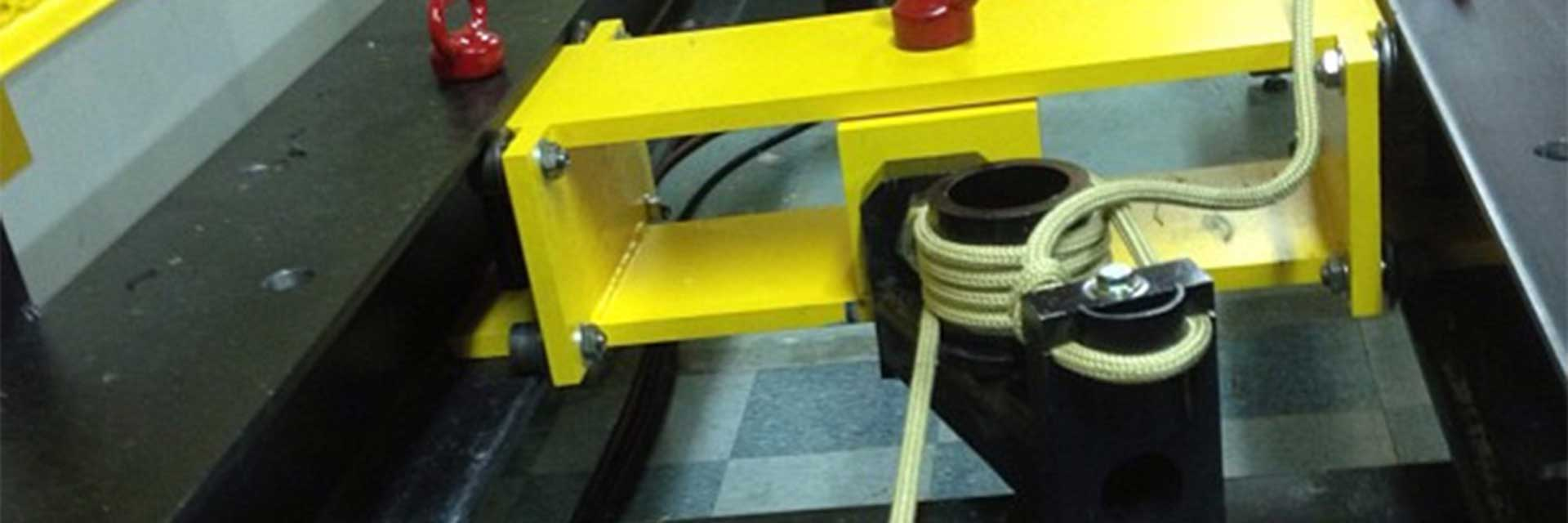# What is 3-Sigma?

Jim Ewing, Sterling Technical Advisor Oct 13th 2017• 3-sigma testing, determines the minimum break strength (mbs) of our ropes

Sterling Rope uses 3-sigma testing, also known as the three-sigma rule, to evaluate and determine the minimum break strength (mbs) of our ropes. Three-sigma is a statistical tool used to calculate probability. Minimum break strength is very different from average break strength (abs), although, abs is used in the calculation of mbs.

The three-sigma value is determined by calculating the standard deviation (a complex and tedious calculation on its own) of a series of five breaks. Then multiply that value by three (hence three-sigma) and finally subtract that product from the average of the entire series.  It is 99.73% probable any additional breaks will be at or above this final value.

Sterling applies three-sigma testing for mbs to our standards because it is much more rigorous and therefore safer than using simply the average break strength.

##### The three-sigma process:
1. Carryout at least 5 breaks of the item to be rated
2. Calculate the mean
3. Calculate the standard deviation
4. Multiply the standard deviation by 3
5. Subtract the product in step 4 from the mean

For those of you who are really into math and 3-sigma, there's a good explanation in this video about normal distribution: